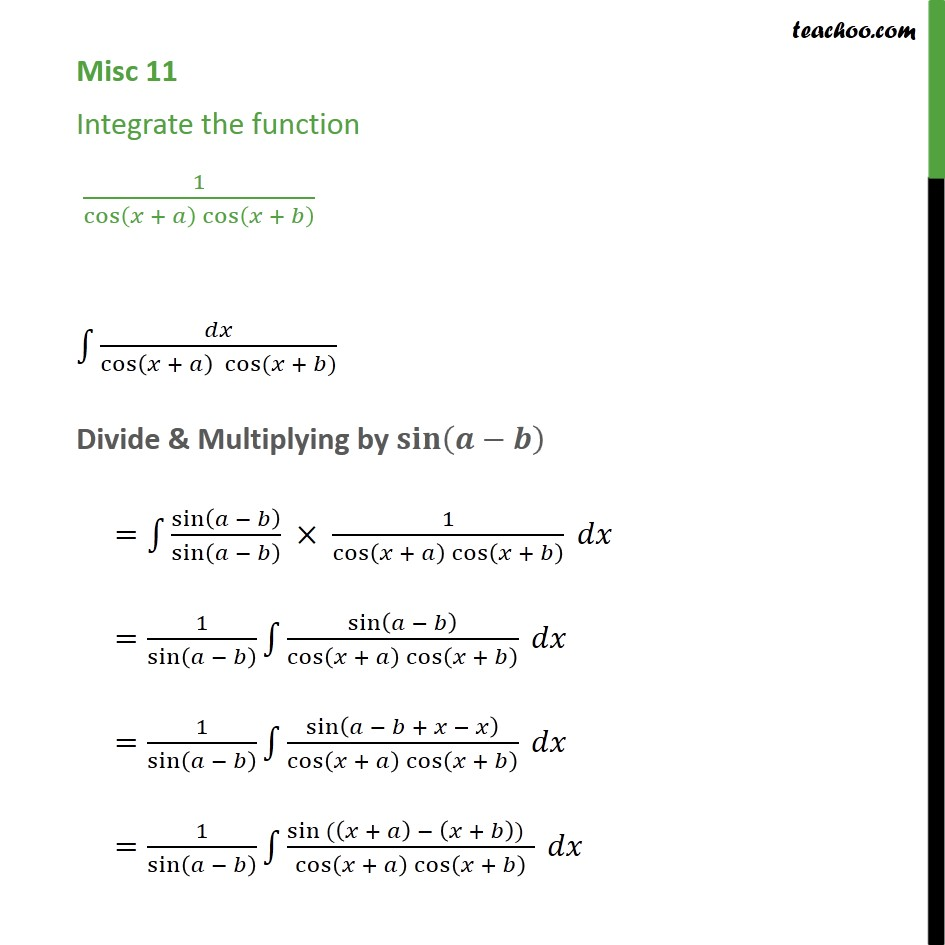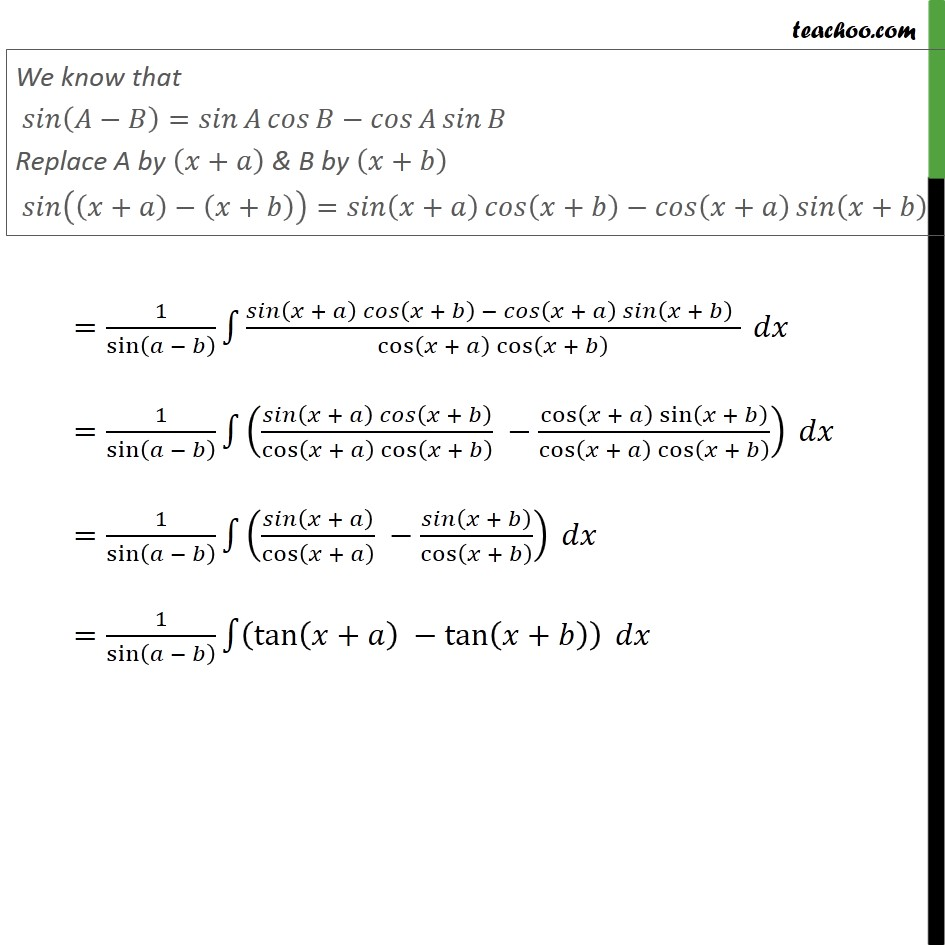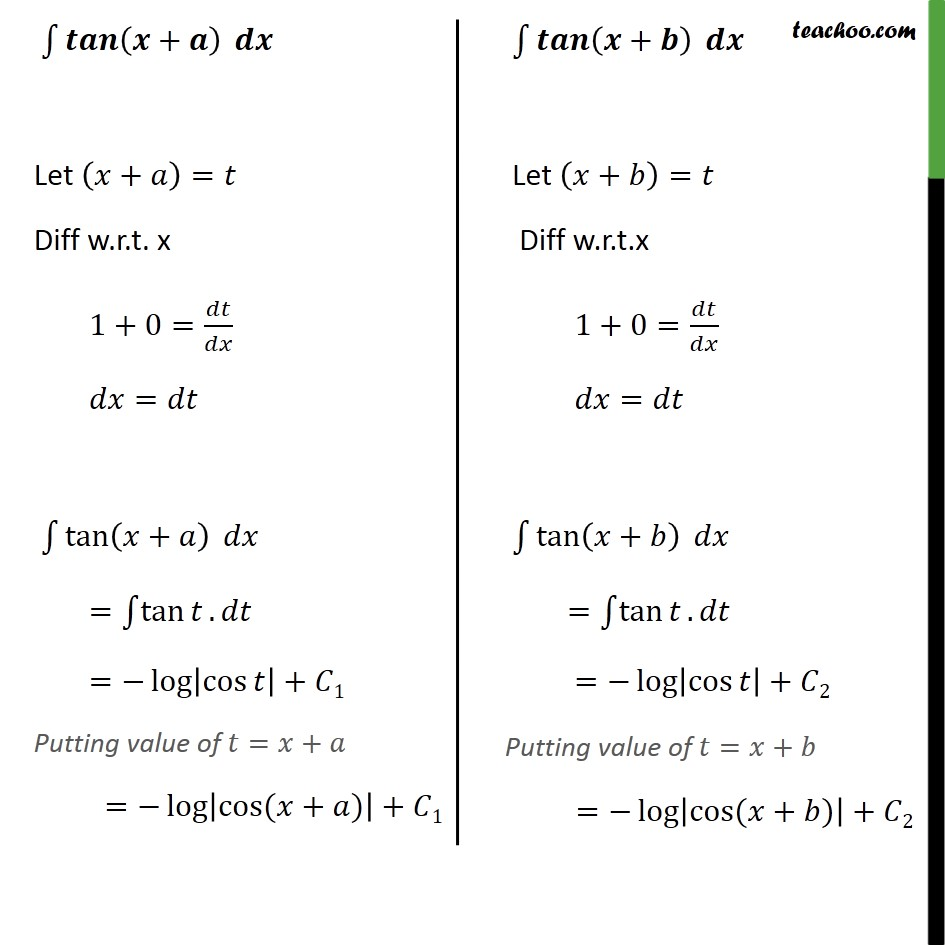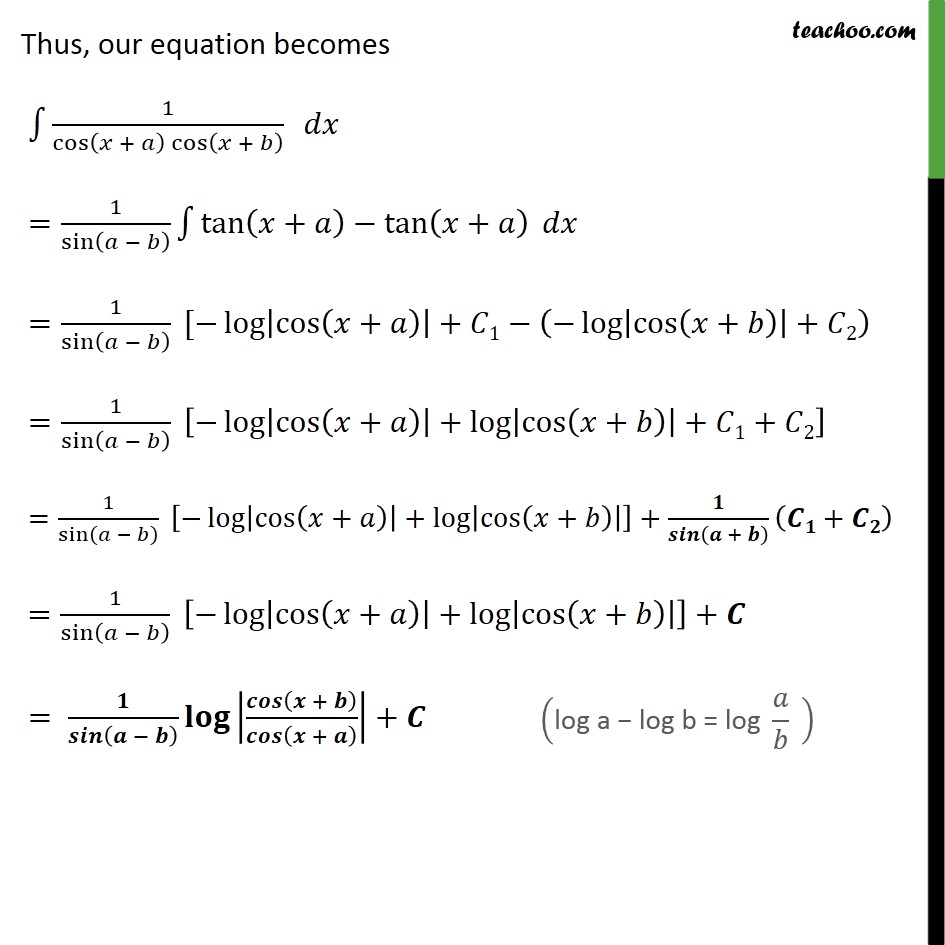Miscellaneous

Chapter 7 Class 12 Integrals
Serial order wiseLearn in your speed, with individual attention - Teachoo Maths 1-on-1 Class

### Transcript

Misc 11 Integrate the function 1/(cos⁡(𝑥 + 𝑎) cos⁡(𝑥 + 𝑏) ) ∫1▒𝑑𝑥/cos⁡〖(𝑥 + 𝑎) cos⁡〖(𝑥 + 𝑏)〗 〗 Divide & Multiplying by 𝐬𝐢𝐧⁡(𝒂−𝒃) =∫1▒〖sin⁡(𝑎 − 𝑏)/sin⁡(𝑎 − 𝑏) × 1/(cos⁡(𝑥 + 𝑎) cos⁡(𝑥 + 𝑏) )〗 𝑑𝑥 =1/sin⁡(𝑎 − 𝑏) ∫1▒sin⁡(𝑎 − 𝑏)/(cos⁡(𝑥 + 𝑎) cos⁡(𝑥 + 𝑏) ) 𝑑𝑥 =1/sin⁡(𝑎 − 𝑏) ∫1▒sin⁡(𝑎 − 𝑏 + 𝑥 − 𝑥)/(cos⁡(𝑥 + 𝑎) cos⁡(𝑥 + 𝑏) ) 𝑑𝑥 =1/sin⁡(𝑎 − 𝑏) ∫1▒(〖sin 〗⁡〖((𝑥 + 𝑎)〗 − (𝑥 + 𝑏)) )/(cos⁡(𝑥 + 𝑎) cos⁡(𝑥 + 𝑏) ) 𝑑𝑥 We know that 𝑠𝑖𝑛⁡(𝐴−𝐵)=𝑠𝑖𝑛⁡𝐴 𝑐𝑜𝑠⁡𝐵−𝑐𝑜𝑠⁡𝐴 𝑠𝑖𝑛⁡𝐵 Replace A by (𝑥+𝑎) & B by (𝑥+𝑏) 𝑠𝑖𝑛⁡((𝑥+𝑎)−(𝑥+𝑏))=𝑠𝑖𝑛⁡(𝑥+𝑎) 𝑐𝑜𝑠⁡(𝑥+𝑏)−𝑐𝑜𝑠⁡(𝑥+𝑎) 𝑠𝑖𝑛⁡(𝑥+𝑏) =1/sin⁡(𝑎 − 𝑏) ∫1▒(𝑠𝑖𝑛⁡(𝑥 + 𝑎) 𝑐𝑜𝑠⁡(𝑥 + 𝑏) − 𝑐𝑜𝑠⁡(𝑥 + 𝑎) 𝑠𝑖𝑛⁡(𝑥 + 𝑏)" " )/(cos⁡(𝑥 + 𝑎) cos⁡(𝑥 + 𝑏) ) 𝑑𝑥 =1/sin⁡(𝑎 − 𝑏) ∫1▒((𝑠𝑖𝑛⁡(𝑥 + 𝑎) 𝑐𝑜𝑠⁡(𝑥 + 𝑏))/(cos⁡(𝑥 + 𝑎) cos⁡(𝑥 + 𝑏) ) −(cos⁡(𝑥 + 𝑎) sin⁡(𝑥 + 𝑏))/(cos⁡(𝑥 + 𝑎) cos⁡(𝑥 + 𝑏) )) 𝑑𝑥 =1/sin⁡(𝑎 − 𝑏) ∫1▒(𝑠𝑖𝑛⁡(𝑥 + 𝑎)/cos⁡(𝑥 + 𝑎) −𝑠𝑖𝑛⁡(𝑥 + 𝑏)/cos⁡(𝑥 + 𝑏) ) 𝑑𝑥 =1/sin⁡(𝑎 − 𝑏) ∫1▒(tan⁡(𝑥+𝑎) −tan⁡(𝑥+𝑏) ) 𝑑𝑥 ∫1▒𝒕𝒂𝒏⁡(𝒙+𝒂) 𝒅𝒙 Let (𝑥+𝑎)=𝑡 Diff w.r.t. x 1+0=𝑑𝑡/𝑑𝑥 𝑑𝑥=𝑑𝑡 ∫1▒tan⁡(𝑥+𝑎) 𝑑𝑥 =∫1▒tan⁡𝑡 . 𝑑𝑡 =−log⁡|cos⁡𝑡 |+𝐶1 Putting value of 𝑡=𝑥+𝑎 =−log⁡|cos⁡〖(𝑥+𝑎)〗 |+𝐶1 ∫1▒𝒕𝒂𝒏⁡(𝒙+𝒃) 𝒅𝒙 Let (𝑥+𝑏)=𝑡 Diff w.r.t.x 1+0=𝑑𝑡/𝑑𝑥 𝑑𝑥=𝑑𝑡 ∫1▒tan⁡(𝑥+𝑏) 𝑑𝑥 =∫1▒tan⁡𝑡 . 𝑑𝑡 =−log⁡|cos⁡𝑡 |+𝐶2 Putting value of 𝑡=𝑥+𝑏 =−log⁡|cos⁡〖(𝑥+𝑏)〗 |+𝐶2 Thus, our equation becomes ∫1▒1/(cos⁡(𝑥 + 𝑎) cos⁡(𝑥 + 𝑏) ) 𝑑𝑥 =1/sin⁡(𝑎 − 𝑏) ∫1▒〖tan⁡(𝑥+𝑎)−tan⁡(𝑥+𝑎) 〗 𝑑𝑥 =1/sin⁡(𝑎 − 𝑏) [−log⁡|cos⁡(𝑥+𝑎) |+𝐶1−(−log⁡|cos⁡(𝑥+𝑏) |+𝐶2) =1/sin⁡(𝑎 − 𝑏) [−log⁡|cos⁡(𝑥+𝑎) |+log⁡|cos⁡(𝑥+𝑏) |+𝐶1+𝐶2] =1/sin⁡(𝑎 − 𝑏) [−log⁡|cos⁡(𝑥+𝑎) |+log⁡|cos⁡(𝑥+𝑏) | ]+𝟏/𝒔𝒊𝒏⁡(𝒂 + 𝒃) (𝑪𝟏+𝑪𝟐) =1/sin⁡(𝑎 − 𝑏) [−log⁡|cos⁡(𝑥+𝑎) |+log⁡|cos⁡(𝑥+𝑏) | ]+𝑪 = 𝟏/𝒔𝒊𝒏⁡(𝒂 − 𝒃) 𝐥𝐨𝐠|𝒄𝒐𝒔⁡(𝒙 + 𝒃)/𝒄𝒐𝒔⁡(𝒙 + 𝒂) |+𝑪 ("log a − log b = log " 𝑎/𝑏 " " )